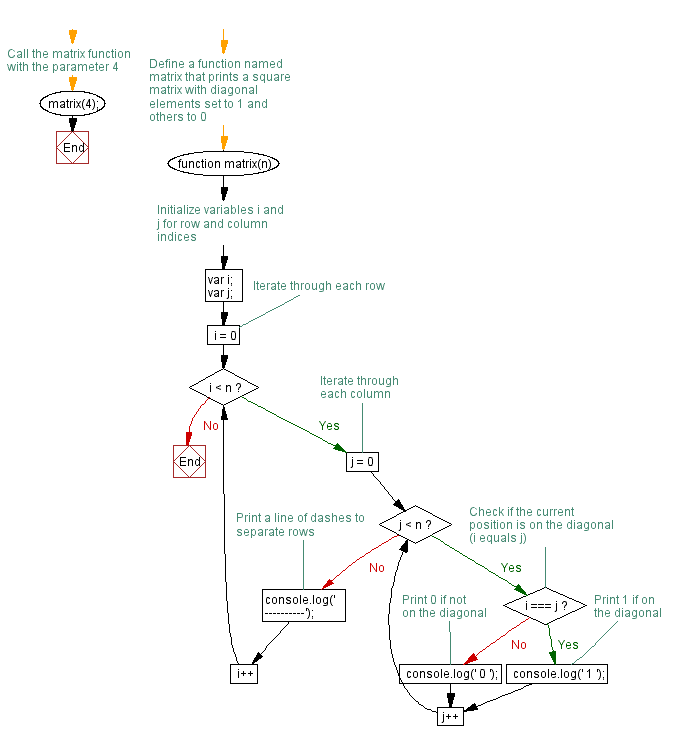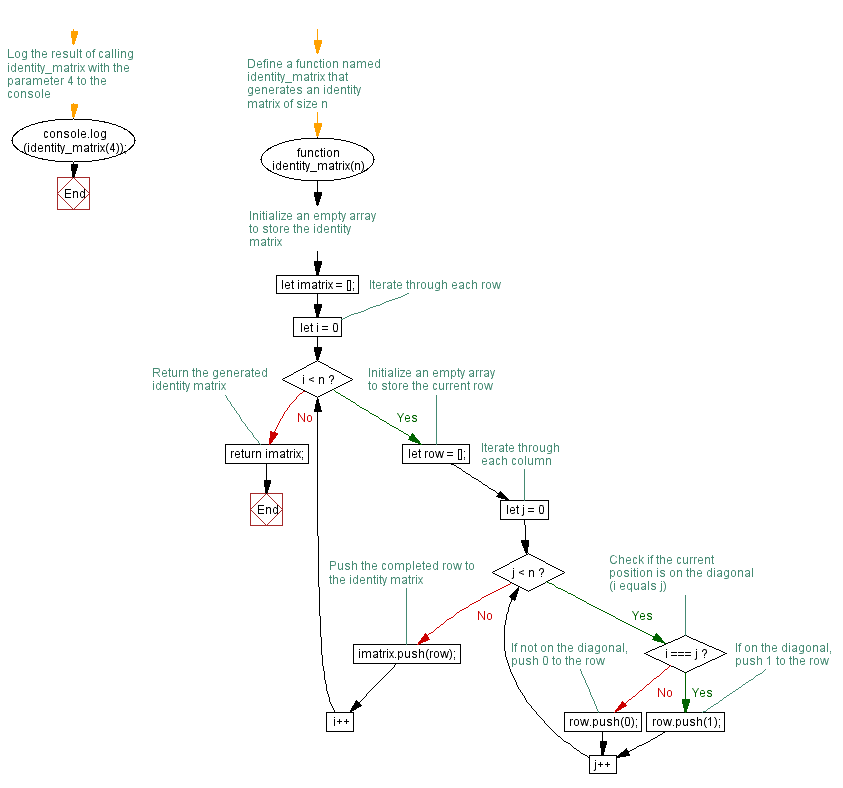# JavaScript: Returns n rows by n columns identity matrix

## JavaScript Function: Exercise-10 with Solution

Write a JavaScript function which returns the n rows by n columns identity matrix.

Pictorial Presentation:Sample Solution-1:

JavaScript Code:

``````function matrix(n) {

var i;
var j;

for (i=0; i < n; i++)
{
for (j=0; j < n; j++)
{
if (i === j)
{

console.log(' 1 ');
}

else
{
console.log(' 0 ');}
}
console.log('----------');
}
}
matrix(4);
```
```

Sample Output:

``` 1
0
0
0
----------
0
1
0
0
----------
0
0
1
0
----------
0
0
0
1
----------

```

Flowchart:Live Demo:

See the Pen JavaScript -Returns n rows by n columns identity matrix-function-ex- 10 by w3resource (@w3resource) on CodePen.

Improve this sample solution and post your code through Disqus

Sample Solution-2:

JavaScript Code:

``````function identity_matrix(n) {
let imatrix = [];
for (let i = 0; i < n; i++) {
let row = [];
for (let j = 0; j < n; j++) {
if (i === j){
row.push(1);
} else {
row.push(0);
}
}
imatrix.push(row);
}
return imatrix;
}
console.log(identity_matrix(4));
```
```

Sample Output:

``` [[1,0,0,0],[0,1,0,0],[0,0,1,0],[0,0,0,1]]
```

Explanation:
The above function first creates an empty matrix array “imatrix“ to hold the identity matrix. It then loops through n times to create n rows and columns. In each row, the array is created empty, and then the loop is repeated n times to create each column. For each column, it checks if the row index i is equal to the column index j. If i and j are equal, it pushes a 1 to the row array, otherwise it pushes a 0. After completing the row, it pushes the row array to the matrix array. Upon creating all rows and columns, the function returns the matrix array representing the identity matrix.

Flowchart:Live Demo:

See the Pen javascript-function-exercise-10-1 by w3resource (@w3resource) on CodePen.

Improve this sample solution and post your code through Disqus

What is the difficulty level of this exercise?

Test your Programming skills with w3resource's quiz.

﻿